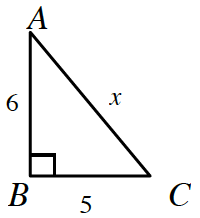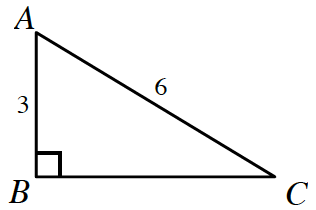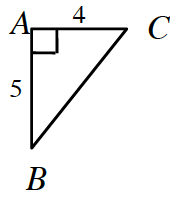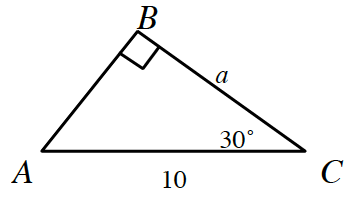### Home > A2C > Chapter 4 > Lesson 4.1.2 > Problem4-30

4-30.

1. $x=$ _______Use the Pythagorean Theorem.

$6^{2}+5^{2}=x^{2}\ \ \ \ \ \ \ \ \ \sqrt{61}=x$

1. $m∠C=$ _______Use the Pythagorean Theorem to find the missing side. Is the triangle a special one?

This is a $30^\circ-60^\circ-90^\circ$ triangle. Therefore, $m∠C$ is $30^\circ$.

1. $m∠B=$ ____The inverse tangent function will be helpful here.

1. $a=$_______Use the cosine ratio or special right triangles.